In general, electric meters can be self-contained or transformer-rated, depending on the amount of load needed. Most homes and some small businesses require the self-contained meter as it can handle smaller load currents and voltages. Businesses, industries and some larger homes require more load and voltages, therefore the transformer-rated meter is used.

## Transformer-Rated Electric Meters

Jackson Energy Authority uses many different variations of transformer-rated electric meter installations as each business, industry and large home requires different amounts of energy to power their needs. Generally speaking, there are three types of transformer-rated electric meter installations: pad mount, cabinet and overhead.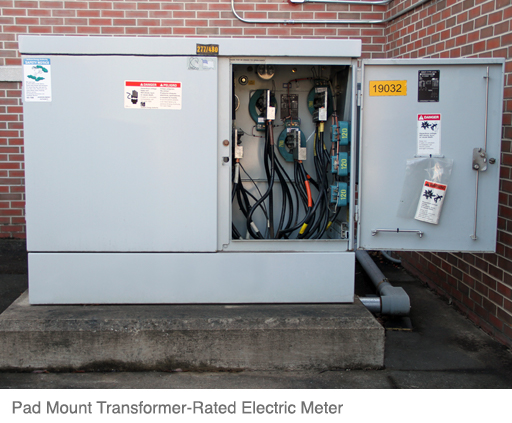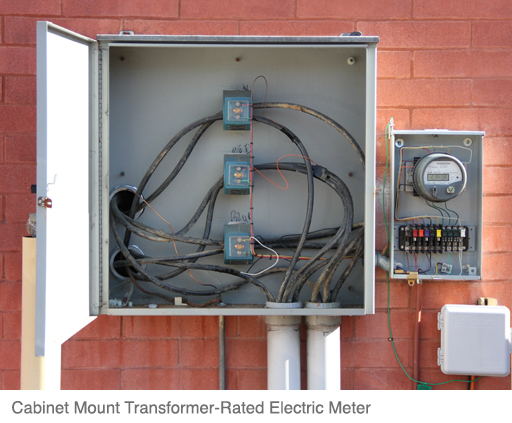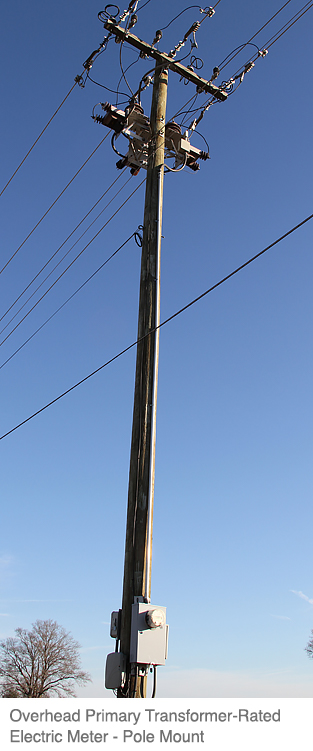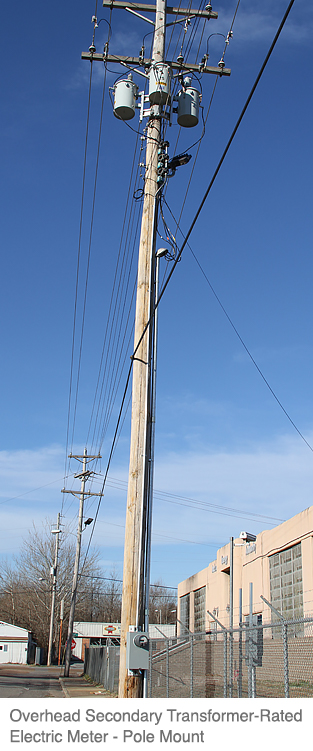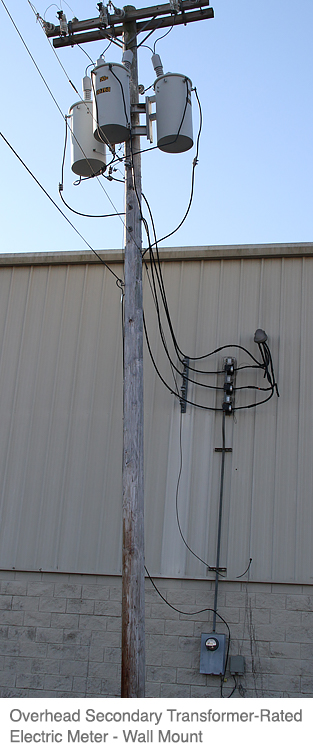The electric service required at the premise is at a current and/or voltage too high for the meter to read. Therefore, each transformer-rated electric meter installation requires Current Transformers (CT) to step down the high current and/or Potential Transformers (PT) to step down high voltage to a safer, more manageable level. The CT’s and PT’s run in parallel with your service, which prevents interruption in service if the meter is removed. For detailed information on meter installations, refer to Meter Bases or the Contractor’s Packet below.

All transformer-rated electric meter installations with CT’s and PT’s step down high current and voltage, which means the meter is only able to measure a fraction of the actual load consumed. This fraction is represented by the engineered ratio of the CT and/or PT, which is the difference between actual consumption and metered consumption. Jackson Energy Authority uses standard size ratios, which are based on the proposed energy usage/demand of the customer.

### Billing the Energy Consumption for Transformer-Rated Electric Meters

To accurately determine usage for billing, a billing multiplier must be used based on the engineered CT/PT ratios. For example:

• A CT with a ratio of 200:5 has been installed. This ratio means that for every 200 amps flowing to the service, the meter will only receive 5 amps. Therefore, the multiplier for this CT is 40.  (200/5=40)
• A 2.4:1 PT has been installed. This ratio means that for every 2.4 volts flowing to the service, the meter will only receive 1 volt. Therefore, the multiplier for this PT is 2.4.  (2.4/1=2.4)
• If the above CT with a multiplier of 40 and PT with a multiplier of 2.4 both have been installed, the two values are multiplied together. Therefore, the multiplier would be 96.  (40x2.4=96)

Once the billing multiplier has been determined, the value is then multiplied by the consumption and demand displayed on the meter. This gives the accurate total for electricity consumption and is the total used when calculating your usage costs.

## Resources

### Contractor Electric Packet

Necessary information for both residential and commercial electric services.